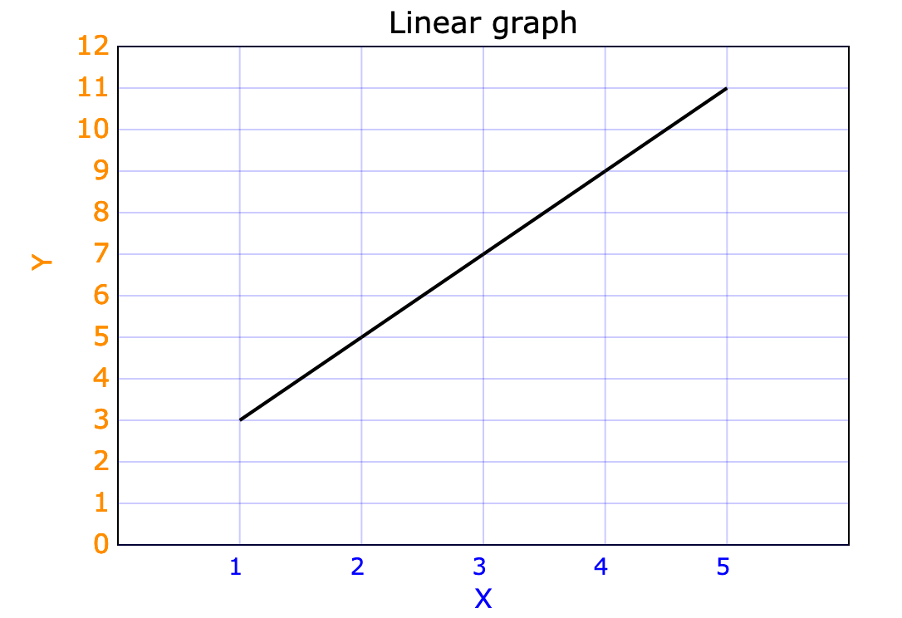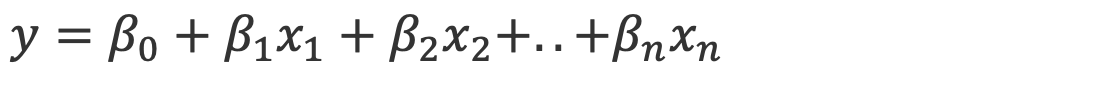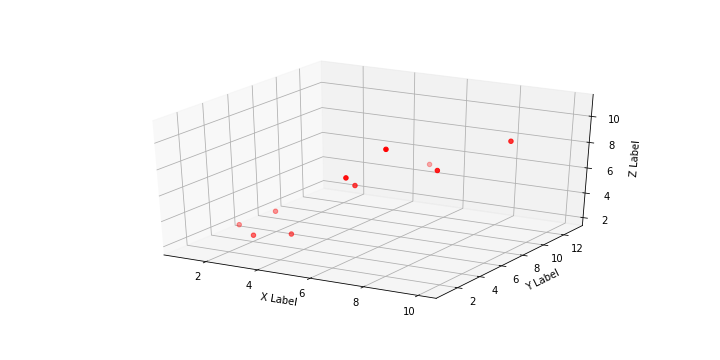# Linear Regression Basics

### Linear Regression Basics

Regression is nothing but establishing a relationship between two or more sets of attributes where the relationship can be between one or multiple independent variables with a dependent variable. In this blog, you will learn about Linear Regression basics and the relationship between multiple attributes.

Take the below example to understand the difference between the dependent variable and the independent variable. Here is a hypothetical example of the house price.

 Area Floor Locality Price 2000 2 City 550000 1000 1 Town 150000

In the above example, the price of the house is dependent upon the area of the house (Area), Number of floors (Floor), and the locality where the house is built. Here the attributes such as Area, Floor, and Locality are called independent variables whereas Price is called dependent variable as Price is dependent upon the other three attributes.

Regression can be described as a process of establishing a relationship based on dependent and independent variables. The output of a regression is a continuous variable e.g. Price of the house.

In this module, we will discuss Linear regression basics and its mechanism.

Linear Regression can be classified broadly into two types;

Simple Linear Regression
Multiple Linear Regression

##### Linear relationship:

First, let’s understand the meaning of a Linear relationship.

A linear relationship is basically a straight-line relationship between two or more variables. A mathematically linear relationship defined as below.

y = mx + c

where
m = slope
c = y-intercept

Let’s look at the below graph where x and y values are defined as a straight line, hence follow a linear relationship.
The equation of the line is y= 2x+1Here, the slope is the measure of the change in y due to change in x. In the case of this example, for every 1unit change in x, y will be changed 2 units.

Real-life Example:
If a person drives 30km/hour for 10 hours, then the person will cover 300km total in 10 hours. This is defined by a linear relation where total distance is linearly dependent upon total hour and speed.

However, real-time problems are based on multiple factors, sometimes in hundreds that affect the outcome. In such scenarios, simple linear regression will not work. Let’s discuss the linear equation for multiple dependent variables.

We will consider the example of the House Price prediction problem where the price is not only dependent upon the area of the house but also price depends upon locality, number of floors, Garden area, swimming pool, number of bathrooms, etc.It is possible to visualize up to two dependent variables as below and in this case, the regression line will be a 3D plane.As we cannot plot N-Dimension but still we can extrapolate for N-Dimension mathematically and the regression plane will be of N-Dimension.

Conclusion:

In this blog, you understood the Linear Regression basics, and in the next blog, we will find more about Simple Linear Regression and the best-fit line.